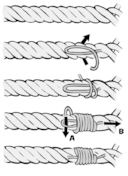Want to share your content on R-bloggers? click here if you have a blog, or here if you don't.Binding vectors, matrices and data frames using `rbind` and `cbind` is a common R task. However, when dimensions or classes differ between the objects passed to these functions, errors or unexpected results are common as well. Sounds familiar? Time to practice!

Answers to the exercises are available here.

Exercise 1
Try to create matrices from the vectors below, by binding them column-wise. First, without using R, write down whether binding the vectors to a matrix is actually possible; then the resulting matrix and its mode (e.g., character, numeric etc.). Finally check your answer using R.
a. `a <- 1:5 ; b <- 1:5`
b. `a <- 1:5 ; b <- c('1', '2', '3', '4', '5')`
c. `a <- 1:5 ; b <- 1:4; c <- 1:3`

Exercise 2
Repeat exercise 1, binding vectors row-wise instead of column-wise while avoiding any row names.

Exercise 3
Bind the following matrices column-wise. First, without using R, write down whether binding the matrices is actually possible; then the resulting matrix and its mode (e.g., character, numeric etc.). Finally check your answer using R.
a. `a <- matrix(1:12, ncol=4); b <- matrix(21:35, ncol=5)`
b. `a <- matrix(1:12, ncol=4); b <- matrix(21:35, ncol=3)`
c. `a <- matrix(1:39, ncol=3); b <- matrix(LETTERS, ncol=2)`

Exercise 4
Bind the matrix `a <- matrix(1:1089, ncol=33)` to itself, column-wise, 20 times (i.e., resulting in a new matrix with 21*33 columns). Hint: Avoid using `cbind()` to obtain an efficient solution. Various solutions are possible. If yours is different from those shown on the solutions page, please post yours on that page as comment, so we can all benefit.

Exercise 5
Try to create new data frames from the data frames below, by binding them column-wise. First, without using R, write down whether binding the data frames is actually possible; then the resulting data frame and the class of each column (e.g., integer, character, factor etc.). Finally check your answer using R.
a. `a <- data.frame(v1=1:5, v2=LETTERS[1:5]) ; b <- data.frame(var1=6:10, var2=LETTERS[6:10])`
b. `a <- data.frame(v1=1:6, v2=LETTERS[1:6]) ; b <- data.frame(var1=6:10, var2=LETTERS[6:10])`

Exercise 6
Try to create new data frames from the data frames below, by binding them row-wise. First, without using R, write down whether binding the data frames is actually possible; then the resulting data frame and the class of each column (e.g., integer, character, factor etc.). Finally check your answer using R, and explain any unexpected output.
a. `a <- data.frame(v1=1:5, v2=LETTERS[1:5]) ; b <- data.frame(v1=6:10, v2=LETTERS[6:10])`
b. `a <- data.frame(v1=1:6, v2=LETTERS[1:6]) ; b <- data.frame(v2=6:10, v1=LETTERS[6:10])`

Exercise 7
a. Use `cbind()` to add vector `v3 <- 1:5` as a new variable to the data frame created in exercise 6b.
b. Reorder the columns of this data frame, as follows: v1, v3, v2.

Exercise 8
Consider again the matrices of exercise 3b. Use both `cbind()` and `rbind()` to bind both matrices column-wise, adding `NA` for empty cells.

Exercise 9
Consider again the data frames of exercise 5b. Use both `cbind()` and `rbind()` to bind both matrices column-wise, adding `NA` for empty cells.

Image: By Hella, Handdrawing 1995.